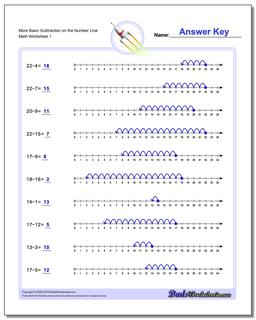# Math Worksheets: Subtraction: Subtraction: More Basic Subtraction on the Number Line## More Basic Subtraction on the Number Line

PropertyValue
DescriptionMore Basic Subtraction on the Number Line: These simple subtraction worksheets introduce subtraction concepts using the number Line.
Resource TypeWorksheet